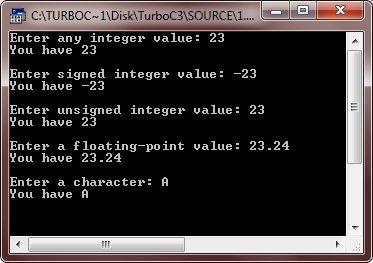# C++ Variable Types

In C++, a variable is basically a named storage that our programs can manipulate. Each and every variable in C++ has particular type, determines the layout and size of the memory of the variable, and the value's range that can be stored within that memory.

## C++ Variable Type Example

Here is an example, uses many types of variables in C++:

```/* C++ Variable Types */

#include<iostream.h>
#include<conio.h>
void main()
{
clrscr();

int i;
float f;
char c;
double d;
signed int si;
unsigned int ui;

cout<<"Enter any integer value: ";
cin>>i;
cout<<"You have "<<i;
cout<<"\n\nEnter signed integer value: ";
cin>>si;
cout<<"You have "<<si;
cout<<"\n\nEnter unsigned integer value: ";
cin>>ui;
cout<<"You have "<<ui;
cout<<"\n\nEnter a floating-point value: ";
cin>>f;
cout<<"You have "<<f;
cout<<"\n\nEnter a character: ";
cin>>c;
cout<<"You have "<<c;

getch();
}```

Here is the sample run of this C++ program:C++ also allows to define various other types of variables, which we will cover in subsequent chapters like pointers, arrays, references, structures, and class, etc.

### More Examples

Here are some more C++ programs listed, that you may like:

Tools
Calculator

Quick Links
Signup - Login - Give Online Test